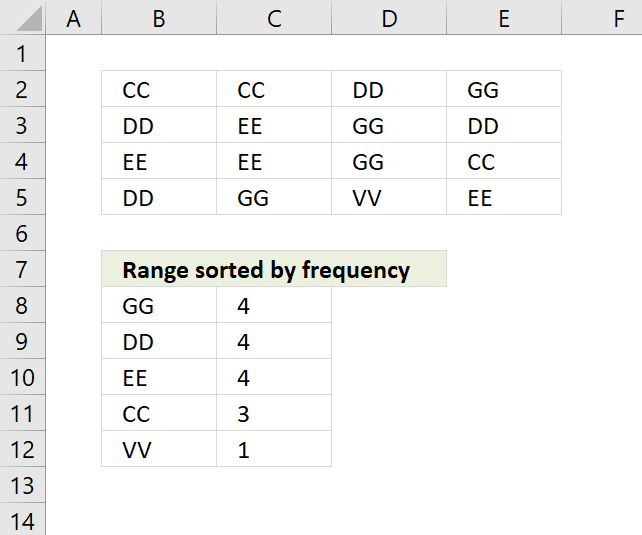Author: Oscar Cronquist Article last updated on November 28, 2018The formula in cell B8 extracts a list sorted based on frequency.

Array formula in B8:

=TEXTJOIN("", TRUE, IF(MIN(IF((MAX(IF(COUNTIF(\$B\$7:B7, \$B\$2:\$E\$5)=0, COUNTIF(\$B\$2:\$E\$5, \$B\$2:\$E\$5), ""))=IF(COUNTIF(\$B\$2:\$E\$5, \$B\$2:\$E\$5)*(COUNTIF(\$B\$7:B7, \$B\$2:\$E\$5)=0), COUNTIF(\$B\$2:\$E\$5, \$B\$2:\$E\$5)*(COUNTIF(\$B\$7:B7, \$B\$2:\$E\$5)=0), "")), (ROW(\$B\$2:\$E\$5)+(1/(COLUMN(\$B\$2:\$E\$5)+1)))*1, ""))=(ROW(\$B\$2:\$E\$5)+(1/(COLUMN(\$B\$2:\$E\$5)+1))*1), \$B\$2:\$E\$5, ""))

copied down as far as necessary.

### Explaining formula in cell B8

#### Step 1 - Count previous values

The COUNTIF function counts values based on a condition or criteria. The first argument \$B\$7:B7 expands when the cell is copied to cells below.

COUNTIF(\$B\$7:B7, tbl)=0

becomes

{0,0,0,0;0,0,0,0;0,0,0,0;0,0,0,0}=0

and returns

{TRUE, TRUE, TRUE, TRUE; TRUE, TRUE, TRUE, TRUE; TRUE, TRUE, TRUE, TRUE; TRUE, TRUE, TRUE, TRUE}

#### Step 2 - Replace TRUE with frequency count

The IF function returns the corresponding frequency number if boolean value is TRUE. FALSE returns "" (nothing).

IF(COUNTIF(\$B\$7:B7,\$B\$2:\$E\$5)=0,COUNTIF(\$B\$2:\$E\$5,\$B\$2:\$E\$5),"")

becomes

IF({TRUE, TRUE, TRUE, TRUE; TRUE, TRUE, TRUE, TRUE; TRUE, TRUE, TRUE, TRUE; TRUE, TRUE, TRUE, TRUE}, {3,3,4,4;4,4,4,4;4,4,4,3;4,4,1,4},"")

and returns

{3,3,4,4;4,4,4,4;4,4,4,3;4,4,1,4}.

#### Step 3 - Find largest value in array

The MAX function returns the maximum number in array ignoring blanks and text values.

MAX(IF(COUNTIF(\$B\$7:B7, \$B\$2:\$E\$5)=0, COUNTIF(\$B\$2:\$E\$5, \$B\$2:\$E\$5), ""))

becomes

MAX({3,3,4,4;4,4,4,4;4,4,4,3;4,4,1,4})

and returns 4.

#### Step 4 - Compare the largest number to array

IF((MAX(IF(COUNTIF(\$B\$7:B7, \$B\$2:\$E\$5)=0, COUNTIF(\$B\$2:\$E\$5, \$B\$2:\$E\$5), ""))=IF(COUNTIF(\$B\$2:\$E\$5, \$B\$2:\$E\$5)*(COUNTIF(\$B\$7:B7, \$B\$2:\$E\$5)=0), COUNTIF(\$B\$2:\$E\$5, \$B\$2:\$E\$5)*(COUNTIF(\$B\$7:B7, \$B\$2:\$E\$5)=0), "")), (ROW(\$B\$2:\$E\$5)+(1/(COLUMN(\$B\$2:\$E\$5)+1)))*1, "")

becomes

IF(4=IF(COUNTIF(\$B\$2:\$E\$5, \$B\$2:\$E\$5)*(COUNTIF(\$B\$7:B7, \$B\$2:\$E\$5)=0), COUNTIF(\$B\$2:\$E\$5, \$B\$2:\$E\$5)*(COUNTIF(\$B\$7:B7, \$B\$2:\$E\$5)=0), "")), (ROW(\$B\$2:\$E\$5)+(1/(COLUMN(\$B\$2:\$E\$5)+1)))*1, "")

becomes

IF(4={3,3,4,4;4,4,4,4;4,4,4,3;4,4,1,4}, (ROW(\$B\$2:\$E\$5)+(1/(COLUMN(\$B\$2:\$E\$5)+1)))*1, "")

becomes

IF(4={3,3,4,4;4,4,4,4;4,4,4,3;4,4,1,4}, {2.33333333333333, 2.25, 2.2, 2.16666666666667;3.33333333333333, 3.25, 3.2, 3.16666666666667;4.33333333333333, 4.25, 4.2, 4.16666666666667;5.33333333333333, 5.25, 5.2, 5.16666666666667}, "")

and returns

{"", "", 2.2, 2.16666666666667;3.33333333333333, 3.25, 3.2, 3.16666666666667;4.33333333333333, 4.25, 4.2, "";5.33333333333333, 5.25, "", 5.16666666666667}.

#### Step 5 - Replace TRUE with unique number

IF(MIN(IF((MAX(IF(COUNTIF(\$B\$7:B7, \$B\$2:\$E\$5)=0, COUNTIF(\$B\$2:\$E\$5, \$B\$2:\$E\$5), ""))=IF(COUNTIF(\$B\$2:\$E\$5, \$B\$2:\$E\$5)*(COUNTIF(\$B\$7:B7, \$B\$2:\$E\$5)=0), COUNTIF(\$B\$2:\$E\$5, \$B\$2:\$E\$5)*(COUNTIF(\$B\$7:B7, \$B\$2:\$E\$5)=0), "")), (ROW(\$B\$2:\$E\$5)+(1/(COLUMN(\$B\$2:\$E\$5)+1)))*1, ""))=(ROW(\$B\$2:\$E\$5)+(1/(COLUMN(\$B\$2:\$E\$5)+1))*1), \$B\$2:\$E\$5, "")

becomes

IF(MIN({"", "", 2.2, 2.16666666666667;3.33333333333333, 3.25, 3.2, 3.16666666666667;4.33333333333333, 4.25, 4.2, "";5.33333333333333, 5.25, "", 5.16666666666667})=(ROW(\$B\$2:\$E\$5)+(1/(COLUMN(\$B\$2:\$E\$5)+1))*1), \$B\$2:\$E\$5, "")

becomes

IF(2.16666666666667=(ROW(\$B\$2:\$E\$5)+(1/(COLUMN(\$B\$2:\$E\$5)+1))*1), \$B\$2:\$E\$5, "")

becomes

IF(2.16666666666667={2.33333333333333, 2.25, 2.2, 2.16666666666667;3.33333333333333, 3.25, 3.2, 3.16666666666667;4.33333333333333, 4.25, 4.2, 4.16666666666667;5.33333333333333, 5.25, 5.2, 5.16666666666667}, \$B\$2:\$E\$5, "")

becomes

IF({FALSE, FALSE, FALSE, TRUE; FALSE, FALSE, FALSE, FALSE; FALSE, FALSE, FALSE, FALSE; FALSE, FALSE, FALSE, FALSE}, \$B\$2:\$E\$5, "")

becomes

IF({FALSE, FALSE, FALSE, TRUE; FALSE, FALSE, FALSE, FALSE; FALSE, FALSE, FALSE, FALSE; FALSE, FALSE, FALSE, FALSE}, {"CC", "CC", "DD", "GG";"DD", "EE", "GG", "DD";"EE", "EE", "GG", "CC";"DD", "GG", "VV", "EE"}, "")

and returns

{"","","","GG";"","","","";"","","","";"","","",""}.

#### Step 6 - Concatenate strings in array

The TEXTJOIN function returns values concatenated ignoring blanks in array.

TEXTJOIN("", TRUE, IF(MIN(IF((MAX(IF(COUNTIF(\$B\$7:B7, \$B\$2:\$E\$5)=0, COUNTIF(\$B\$2:\$E\$5, \$B\$2:\$E\$5), ""))=IF(COUNTIF(\$B\$2:\$E\$5, \$B\$2:\$E\$5)*(COUNTIF(\$B\$7:B7, \$B\$2:\$E\$5)=0), COUNTIF(\$B\$2:\$E\$5, \$B\$2:\$E\$5)*(COUNTIF(\$B\$7:B7, \$B\$2:\$E\$5)=0), "")), (ROW(\$B\$2:\$E\$5)+(1/(COLUMN(\$B\$2:\$E\$5)+1)))*1, ""))=(ROW(\$B\$2:\$E\$5)+(1/(COLUMN(\$B\$2:\$E\$5)+1))*1), \$B\$2:\$E\$5, ""))

becomes

TEXTJOIN("", TRUE, {"","","","GG";"","","","";"","","","";"","","",""})

and returns "GG" in cell B8.